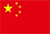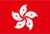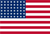Scanning QR Code• Online Inquiry

+
Congratulations
You've successfully got advanced quotes worth 5000 HKD
• Home
• Quotes
• News
• Learn
• Help Center
• Language
•••EN

# KDJ

## 1. Introduction

Stochastic indicators were first used in the analysis of the futures market, and then widely used in the shortto mid-term trend analysis of the stock market. Through a specific period (usually 9 days, 9 weeks, etc.), the highest price, the lowest price, and the closing price of the last calculation period and the proportional relationship between these three are used to calculate the last calculation period's RSV(Row Stochastic Value), then calculate the K value, D value and J value according to the method of the smooth moving average, and draw a graph to study the stock trend.

## 2. Calculation formula

The KDJ indicator is to calculate the overbought and oversold scenarioin n days. First, calculate the RSV value over aperiod (n days, n weeks, etc.), that is, the immature stochastic index value, and then calculate the K value, D value, J value, etc. Generally, n takes 9 days.

RSV (n days)=(Cn－Ln)/(Hn－Ln)×100

Cn is the closing price on the n-th day; Ln is the lowest price in n days; Hn is the highest price in n days.

Second, calculate the K value and D value:

K value = 2/3 × K value of the previous day + 1/3 × RSV of the day

D value = 2/3 × D value of the previous day + 1/3 × K value of the day

J value = 3 × K value of the day-2 × D value of the day

## 3. Application

3.1 K line is a quick confirming line -a value above 90 is overbought, and a value below 10 is oversold;

3.2 D line is a slow majorline-a value above 80 is overbought, and a value below 20 is oversold;

3.3 J line is a directional sensitive line. When the J value is greater than 90, especially for more than 5 consecutive days, the stock price will at least form a short-term peak. On the contrary, when the J value is less than 10, especially for several consecutive days, the stock price will at least form a short-term bottom.

3.4 When the K line breaks above D line on the graph, it is commonly known as the golden cross, which is a signal to buy.

3.5 Inapractical trading activity, when the K and D lines cross upwards below 20, the short-term buy signal at this time is more accurate; if the K value is below 50, crossing above the D value twice, forming a higher high golden cross「W」 pattern, the stock price may have a considerable increase in the market outlook.

3.6 When the K value is diminishing then break belowD line from above, it is commonly called a dead cross. That is the signal to sell.

3.7 Inapractical trading activity, when the K and D lines cross down over an 80 level, the short-term selling signal at this time is more accurate; if the K value is above 50, crossing below the D line twice in a trend and froma lower low death cross "M" pattern, the market outlook may have a considerable decline in stock prices.

3.8 It is also a practical method to gauge the peakand bottom of the stock price based on the trend of the deviation between KDJ and the stock price:

● The stock price has hit a new high, but the KD value has not hit a new high could be considered as a divergence from the top, it should be sold;

● The stock price has hit a new low, but the KD value has not hit a new low, which is a divergence from the bottom and it should be bought;

● The stock price did not hit a new high, but the KD value hit a new high, which is a top divergence and should be sold;

● The stock price has not hit a new low, but the KD value has hit a new low, which is a bottom divergence and should be bought.

3.9 It should be noted that the method of determining the top and bottom divergenceof KDJ can only be compared with the KD value of the previous trendof high and low, and cannot be gapped a session over.

Back to the Top